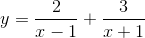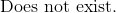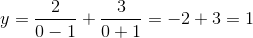# SAT II Math I : X-intercept and y-intercept

## Example Questions

2 Next →

### Example Question #31 : Functions And Graphs

What is the y-intercept of the function?Explanation:

The y-intercept is the value ofwhen.

Substitute zero into the x-variable in the equation.The y-intercept is.

The answer is:2 Next →